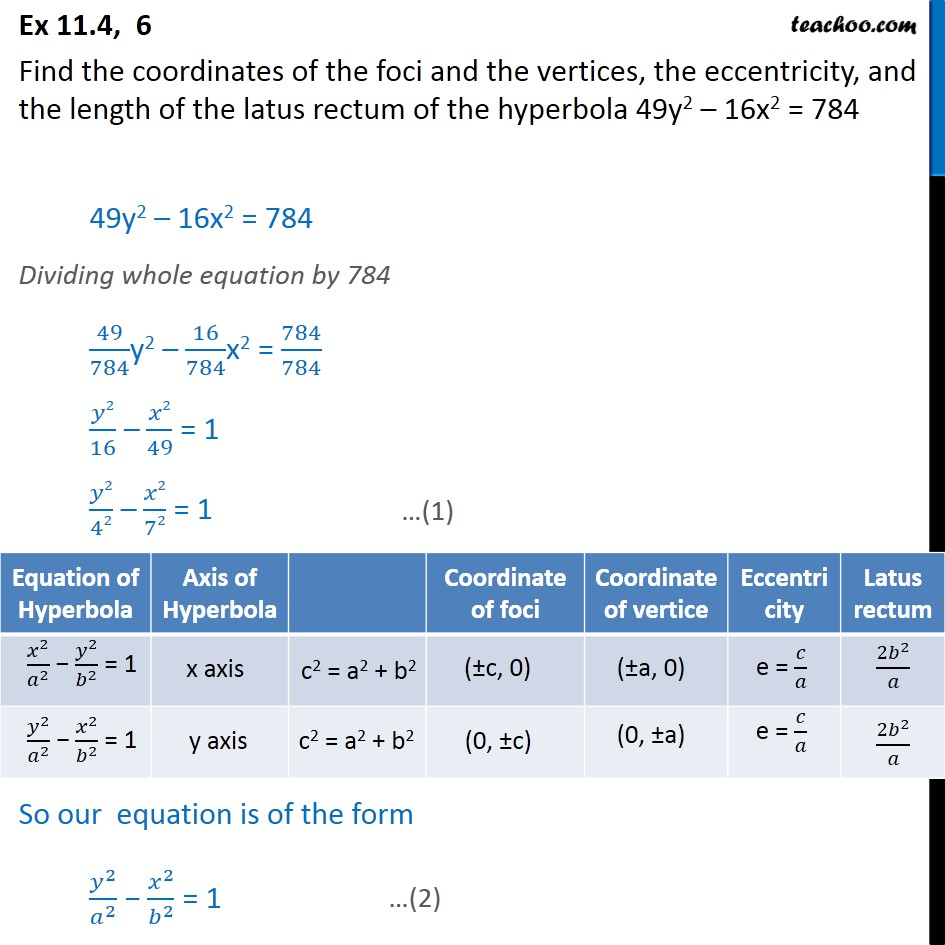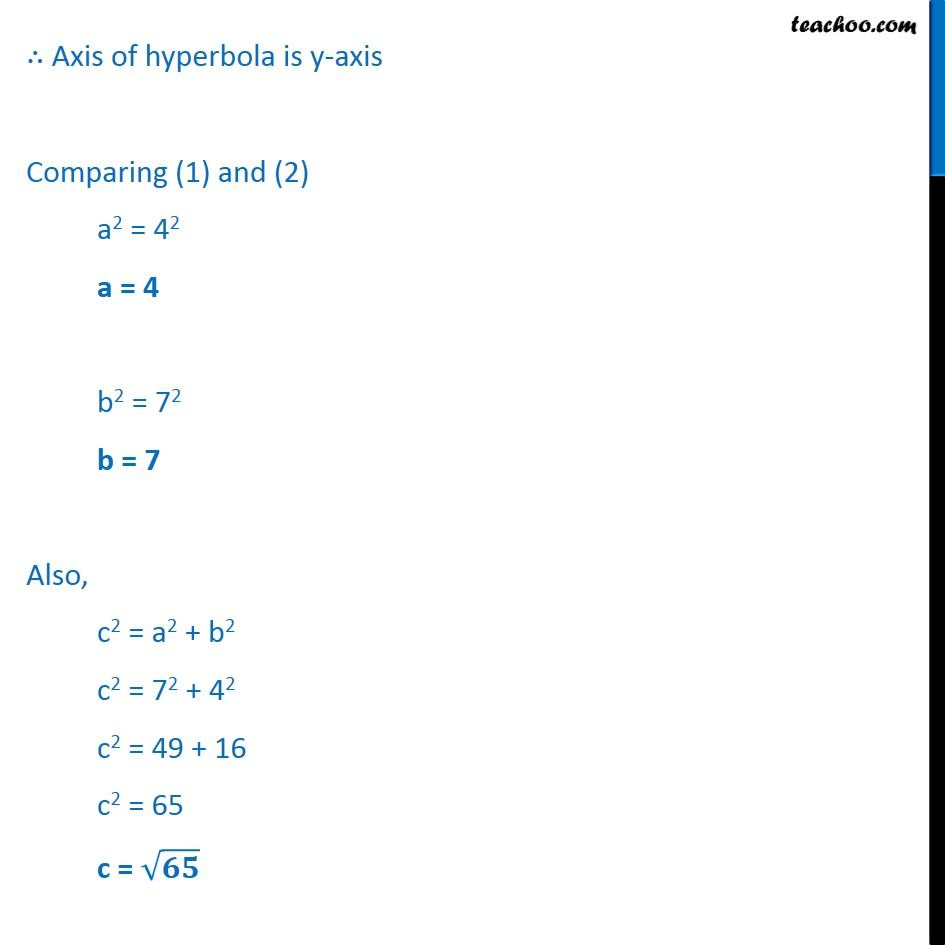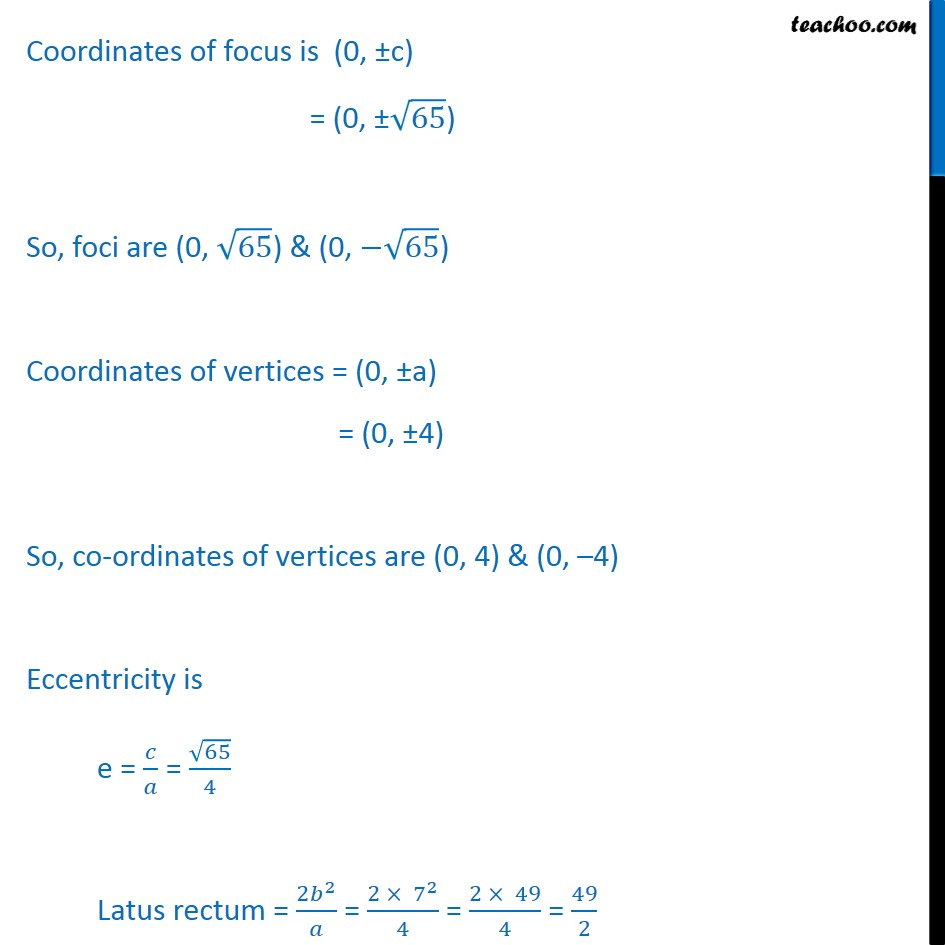Ex 10.4

Chapter 10 Class 11 Conic Sections
Serial order wiseLearn in your speed, with individual attention - Teachoo Maths 1-on-1 Class

### Transcript

Ex 10.4, 6 Find the coordinates of the foci and the vertices, the eccentricity, and the length of the latus rectum of the hyperbola 49y2 16x2 = 784 49y2 16x2 = 784 Dividing whole equation by 784 49 784 y2 16 784 x2 = 784 784 2 16 2 49 = 1 2 42 2 72 = 1 So our equation is of the form 2 2 2 2 = 1 Axis of hyperbola is y-axis Comparing (1) and (2) a2 = 42 a = 4 b2 = 72 b = 7 Also, c2 = a2 + b2 c2 = 72 + 42 c2 = 49 + 16 c2 = 65 c = Coordinates of focus is (0, c) = (0, 65 ) So, foci are (0, 65 ) & (0, 65 ) Coordinates of vertices = (0, a) = (0, 4) So, co-ordinates of vertices are (0, 4) & (0, 4) Eccentricity is e = = 65 4 Latus rectum = 2 2 = 2 7 2 4 = 2 49 4 = 49 2